# Test: Unit Digits, Factorial Powers

## 10 Questions MCQ Test Quantitative Aptitude for GMAT | Test: Unit Digits, Factorial Powers

Description
Attempt Test: Unit Digits, Factorial Powers | 10 questions in 20 minutes | Mock test for GMAT preparation | Free important questions MCQ to study Quantitative Aptitude for GMAT for GMAT Exam | Download free PDF with solutions
QUESTION: 1

### 1727 has a units digit of:

Solution:

When raising a number to a power, the units digit is influenced only by the units digit of that number.  For example 162 ends in a 6 because 62 ends in a 6.

1727 will end in the same units digit as 727.
The units digit of consecutive powers of 7 follows a distinct pattern: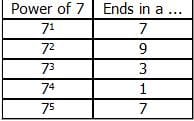The pattern repeats itself every four numbers so a power of 27 represents 6 full iterations of the pattern (6 × 4 = 24) with three left over.  The "leftover three" leaves us back on a "3," the third member of the pattern 7, 9, 3, 1.

QUESTION: 2

### 11+22+33+...+1010 is divided by 5. What is the remainder?

Solution:

When a whole number is divided by 5, the remainder depends on the units digit of that number.
Thus, we need to determine the units digit of the number 11+22+33+...+1010. To do so, we need to first determine the units digit of each of the individual terms in the expression as follows: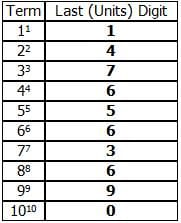To determine the units digit of the expression itself, we must find the sum of all the units digits of each of the individual terms:
1 + 4 + 7 + 6 + 5 + 6 + 3 + 6 + 9 = 47

Thus, 7 is the units digit of the number 11+22+33+...+1010. When an integer that ends in 7 is divided by 5, the remainder is 2. (Test this out on any integer ending in 7.)

Thus, the correct answer is C.

QUESTION: 3

### Given that p is a positive even integer with a positive units digit, if the units digit of p3 minus the units digit of p2 is equal to 0, what is the units digit of p + 3?

Solution:

The easiest way to approach this problem is to chart the possible units digits of the integer p. Since we know p is even, and that the units digit of p is positive, the only options are 2, 4, 6, or 8.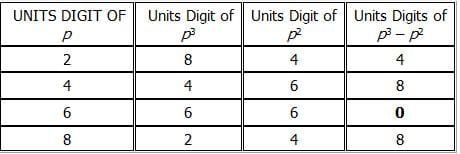Only when the units digit of p is 6, is the units digit of  p3p2 equal to 0.

The question asks for the units digit of p + 3. This is equal to 6 + 3, or 9.

QUESTION: 4

If x is a positive integer, what is the units digit of (24)(2x + 1)(33)(x + 1)(17)(x + 2)(9)(2x)?

Solution:

For problems that ask for the units digit of an expression, yet seem to require too much computation, remember the Last Digit Shortcut. Solve the problem step-by-step, but recognize that you only need to pay attention to the last digit of every intermediate product. Drop any other digits.

So, we can drop any other digits in the original expression, leaving us to find the units digit of:
(4)(2x + 1)(3)(x + 1)(7)(x + 2)(9)(2x)

This problem is still complicated by the fact that we don’t know the value of x. In such situations, it is often a good idea to look for patterns. Let's see what happens when we raise the bases 4, 3, 7, and 9 to various powers. For example: 31 = 3, 32 = 9, 33 = 27, 34 = 81, 35 = 243, and so on. The units digit of the powers of three follow a pattern that repeats every fourth power: 3, 9, 7, 1, 3, 9, 7, 1, and so on. The patterns for the other bases are shown in the table below: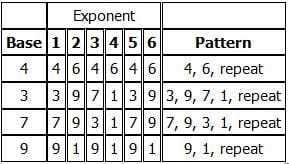The patterns repeat at least every fourth term, so let's find the units digit of (4)(2x + 1)(3)(x + 1)(7)(x + 2)(9)(2x) for at least four consecutive values of x:
x = 1: units digit of (43)(32)(73)(92) = units digit of (4)(9)(3)(1) = units digit of 108 = 8
x = 2: units digit of (45)(33)(74)(94) = units digit of (4)(7)(1)(1) = units digit of 28 = 8
x = 3: units digit of (47)(34)(75)(96) = units digit of (4)(1)(7)(1) = units digit of 28 = 8
x = 4: units digit of (49)(35)(76)(98) = units digit of (4)(3)(9)(1) = units digit of 108 = 8

The units digit of the expression in the question must be 8.

Alternatively, note that x is a positive integer, so 2x is always even, while 2x + 1 is always odd.  Thus,
(4)(2x + 1) = (4)(odd), which always has a units digit of 4
(9)(2x) = (9)(even), which always has a units digit of 1

That leaves us to find the units digit of (3)(x + 1)(7)(x + 2).  Rewriting, and dropping all but the units digit at each intermediate step,
(3)(x + 1)(7)(x + 2)
= (3)(x + 1)(7)(x + 1)(7)
= (3 × 7)(x + 1)(7)
= (21)(x + 1)(7)
= (1)(x + 1)(7) = 7, for any value of x.

So, the units digit of (4)(2x + 1)(3)(x + 1)(7)(x + 2)(9)(2x) is (4)(7)(1) = 28, then once again drop all but the units digit to get 8.

QUESTION: 5

If a and b are positive integers and x = 4a and y = 9b, which of the following is a possible units digit of xy?

Solution:

If a is a positive integer, then 4a will always have a units digit of 4 or 6.  We can show this by listing the first few powers of 4:

41 = 4
42 = 16
43 = 64
44 = 256

The units digit of the powers of 4 alternates between 4 and 6.  Since x = 4a, x will always have a units digit of 4 or 6.

Similarly, if b is a positive integer, then 9b will always have a units digit of 1 or 9.  We can show this by listing the first few powers of 9:

91 = 9
92 = 81
93 = 729
94 = 6561

The units digit of the powers of 9 alternates between 1 and 9.  Since y = 9b, y will always have a units digit of 1 or 9.

To determine the units digit of a product of numbers, we can simply multiply the units digits of the factors.  The resulting units digit is the units digit of the product.  For example, to find the units digit of (23)(39) we can take (3)(9) = 27.  Thus, 7 is the units digit of (23)(39).  So, the units digit of xy will simply be the units digit that results from multiplying the units digit of x by the units digit of y.  Let's consider all the possible units digits of x and y in combination:

4 × 1 = 4, units digit = 4
4 × 9 = 36, units digit = 6
6 × 1 = 6, units digit = 6
6 × 9 = 54, units digit = 4

The units digit of xy will be 4 or 6.

QUESTION: 6

If x = 321 and y = 655, what is the remainder when xy is divided by 10?

Solution:

Since every multiple of 10 must end in zero, the remainder from dividing xy by 10 will be equal to the units’ digit of xy. In other words, the units’ digit will reflect by how much this number is greater than the nearest multiple of 10 and, thus, will be equal to the remainder from dividing by 10. Therefore, we can rephrase the question: “What is the units’ digit of xy?”
Next, let’s look for a pattern in the units’ digit of 321. Remember that the GMAT will not expect you to do sophisticated computations; therefore, if the exponent seems too large to compute, look for a shortcut by recognizing a pattern in the units' digits of the exponent:
31 = 3
32 = 9
33 = 27
34 = 81
35 = 243

As you can see, the pattern repeats every 4 terms, yielding the units digits of 3, 9, 7, and 1. Therefore, the exponents 31, 35, 39, 313, 317, and 321 will end in 3, and the units’ digit of 321 is 3.

Next, let’s determine the units’ digit of 655 by recognizing the pattern:
61 = 6
62 = 36
63 = 256
64 = 1,296

As shown above, all positive integer exponents of 6 have a units’ digit of 6. Therefore, the units' digit of 655 will also be 6.

Finally, since the units’ digit of 321 is 3 and the units’ digit of 655 is 6, the units' digit of 321 × 655 will be equal to 8, since 3 × 6 = 18. Therefore, when this product is divided by 10, the remainder will be 8.

QUESTION: 7

If x is a positive integer, what is the remainder when 712x+3 + 3 is divided by 5?

Solution:

To find the remainder when a number is divided by 5, all we need to know is the units digit, since every number that ends in a zero or a five is divisible by 5.
For example, 23457 has a remainder of 2 when divided by 5 since 23455 would be a multiple of 5, and 23457 = 23455 + 2.
Since we know that x is an integer, we can determine the units digit of the number 712x+3 + 3. The first thing to realize is that this expression is based on a power of 7. The units digit of any integer exponent of seven can be predicted since the units digit of base 7 values follows a patterned sequence: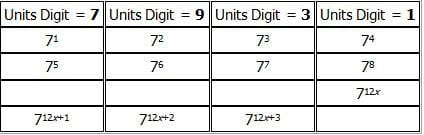We can see that the pattern repeats itself every 4 integer exponents.

The question is asking us about the 12x+3 power of 7. We can use our understanding of multiples of four (since the pattern repeats every four) to analyze the 12x+3 power.

12x is a multiple of 4 since x is an integer, so 712x would end in a 1, just like 74 or 78.
712x+3 would then correspond to 73 or 77 (multiple of 4 plus 3), and would therefore end in a 3.

If 712x+3 ends in a three, 712x+3 + 3 would end in a 3 + 3 = 6.
If a number ends in a 6, there is a remainder of 1 when that number is divided by 5.

QUESTION: 8

What is the units digit of (71)5(46)3(103)4 + (57)(1088)3 ?

Solution:

Since the question only asks about the units digit of the final solution, focus only on computing the units digit for each term. Thus, the question can be rewritten as follows:

(1)5(6)3(3)4 + (7)(8)3.
The units digit of 15 is 1.
The units digit of 63 is 6.
The units digit of 34 is 1.
The units digit of (1 × 6 × 1) is 6.
The units digit of 7 is 7.
The units digit of 83 is 2.
The units digit of (7 × 2) is 4.
The solution is equal to the units digit of (6 + 4), which is 0.

QUESTION: 9

if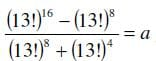, what is the units digit of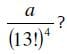Solution:

In order to answer this, we need to recognize a common GMAT pattern: the difference of two squares.  In its simplest form, the difference of two squares can be factored as follows:
x2-y2= (x+y)(x-y)
Where, though, is the difference of two squares in the question above?  It pays to recall that all even exponents are squares.  For example.
x4= (x2)(x2)
x56= (x28)(x28)

Because the numerator in the expression in the question is the difference of two even exponents, we can factor it as the difference of two squares and simplify: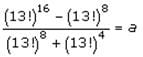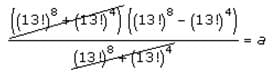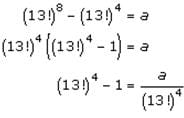The units digit of the left side of the equation is equal to the units digit of the right side of the equation (which is what the question asks about). Thus, if we can determine the units digit of the expression on the left side of the equation, we can answer the question.
Since (13!) = 13 x 12 x 11 x 10 .... x 1, we know that 13! contains a factor of 10, so its units digit must be 0. Similarly, the units digit of (13!)will also have a units digit of 0.  If we subtract 1 from this, we will be left with a number ending in 9.
Therefore, the units digit of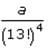is 9.  The correct answer is E.

QUESTION: 10

What is the units digit of 17728 – 13323?

Solution:

Since the question asks only about the units digit, we can look for patterns in each of the numbers.
Let's begin with 17728: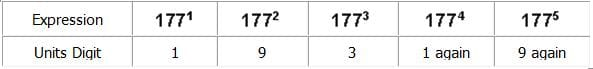Since this pattern will continue, the units digit of 17728 will be 1
Next, let's follow the same procedure with 13323: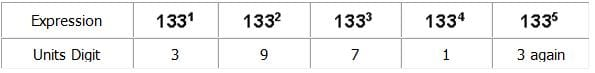Since this pattern will continue, the units digit of 13323 will be 7.
Therefore in calculating the expression 17728-13333 we can determine that the units digit of the solution will equal 1-7
Since, 7 is greater than 1, the subtraction here requires that we carry over from the tens place. Thus, we have 11-7, yielding the units digit 4.Use Code STAYHOME200 and get INR 200 additional OFF Use Coupon Code